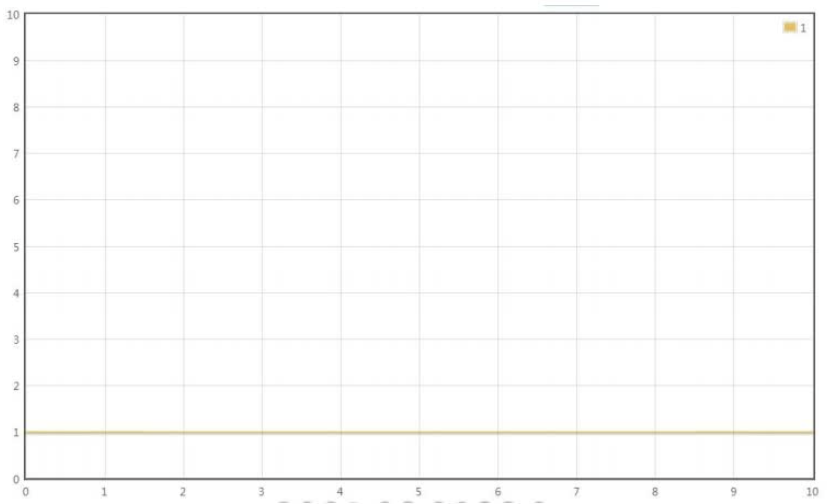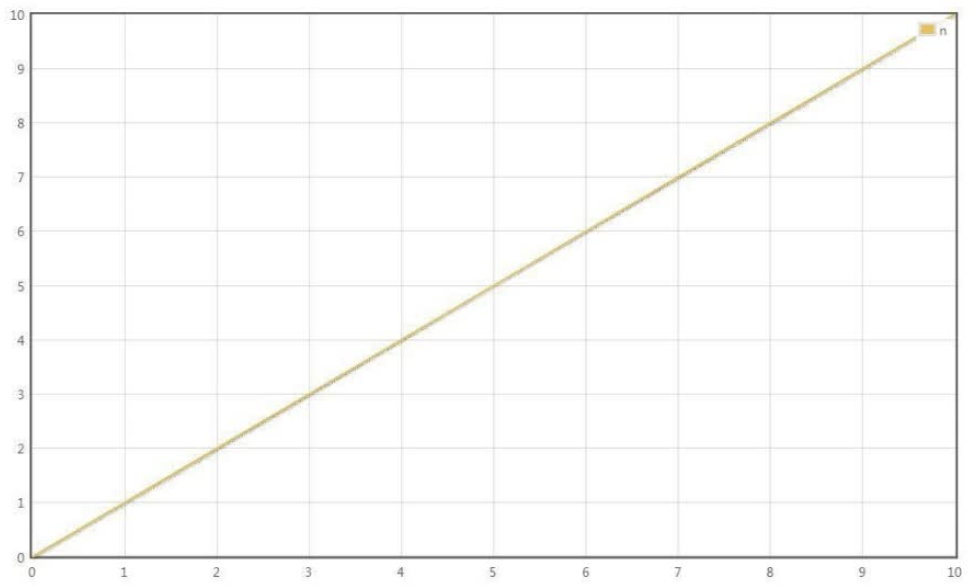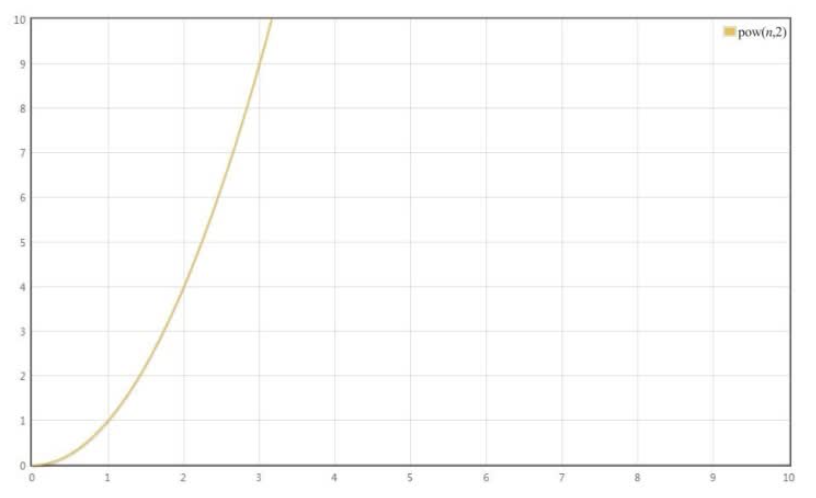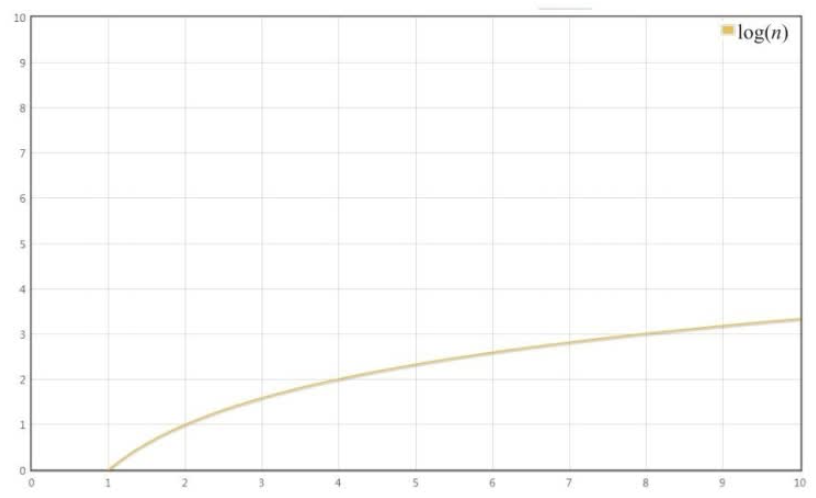• 只保留时间函数中的最高阶项
• 如果运行时间是常数量级的，则用常数 1 表示
• 如果最高阶项存在，则省去最高阶项前面的系数

• $O(1)$• $O(n)$• $O(n^2)$• $O(logn)$

$$\frac{n}{2^k} => 1 => log_2n$$## 参考

• Post title：如何理解时间复杂度
• Post author：Seaman
• Create time：2021-05-20 02:56:47
• Post link：https://gongguowei.com/2021/05/20/time-complexity/
• Copyright Notice：All articles in this blog are licensed under BY-NC-SA unless stating additionally.
Comments